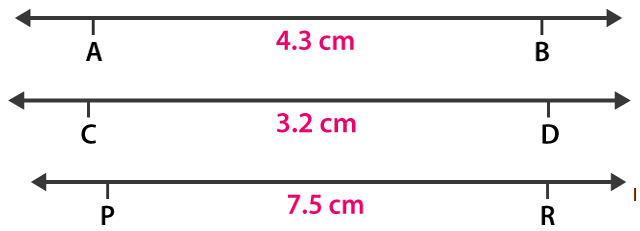# RD Sharma Solutions for Class 6 Maths Chapter 19: Geometrical Constructions Exercise 19.1

In this exercise, we learn about the construction of a line segment of given length with the help of a ruler and compasses. The students can follow the steps mentioned in the solutions’ PDF to gain a better idea of solving problems. The steps of each solution are explained in a simple language to boost the exam preparation of students. In order to understand the concepts and perform well in the exam, students can easily access RD Sharma Solutions Class 6 Maths Chapter 19 Geometrical Constructions Exercise 19.1 PDF from the links which are provided here.

## RD Sharma Solutions for Class 6 Maths Chapter 19: Geometrical Constructions Exercise 19.1 Download PDF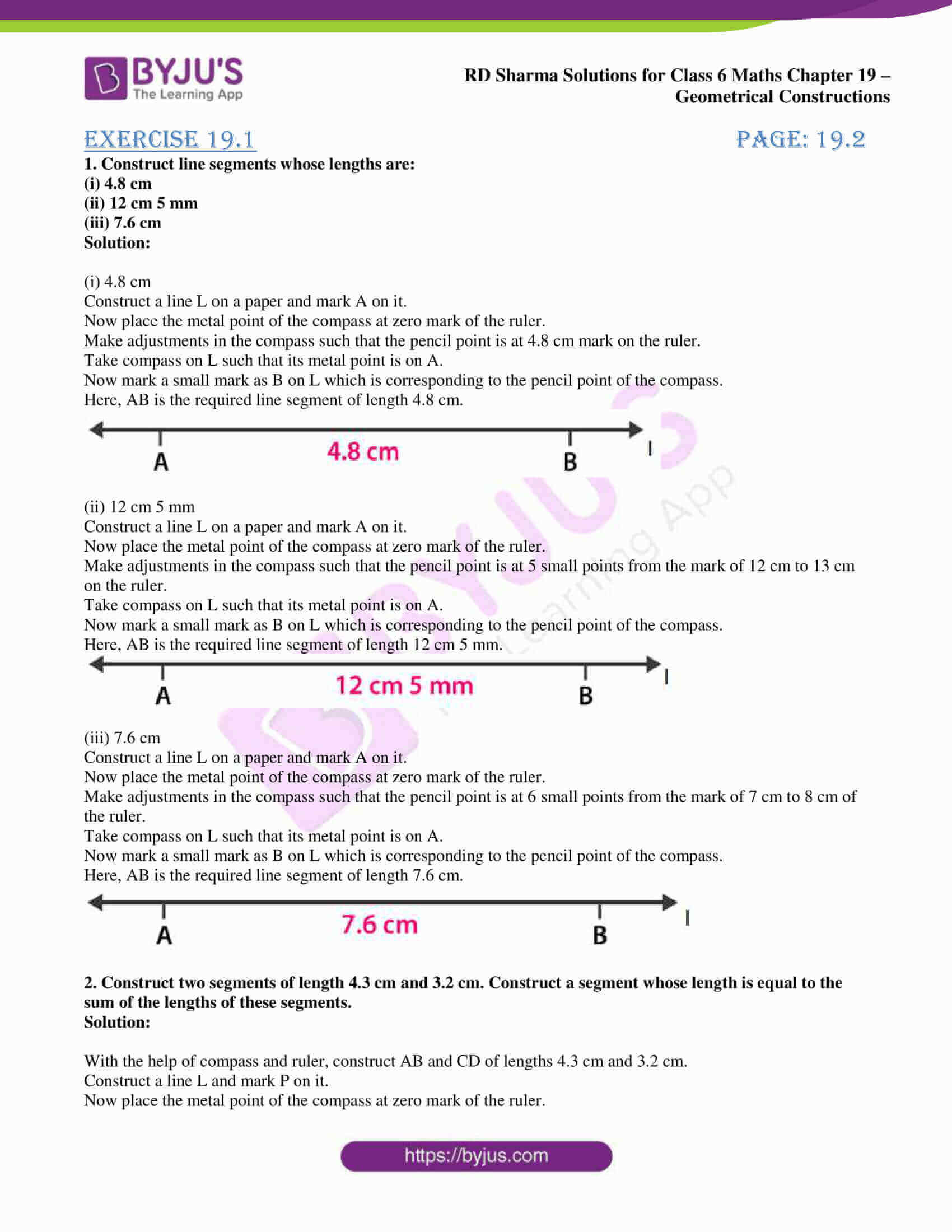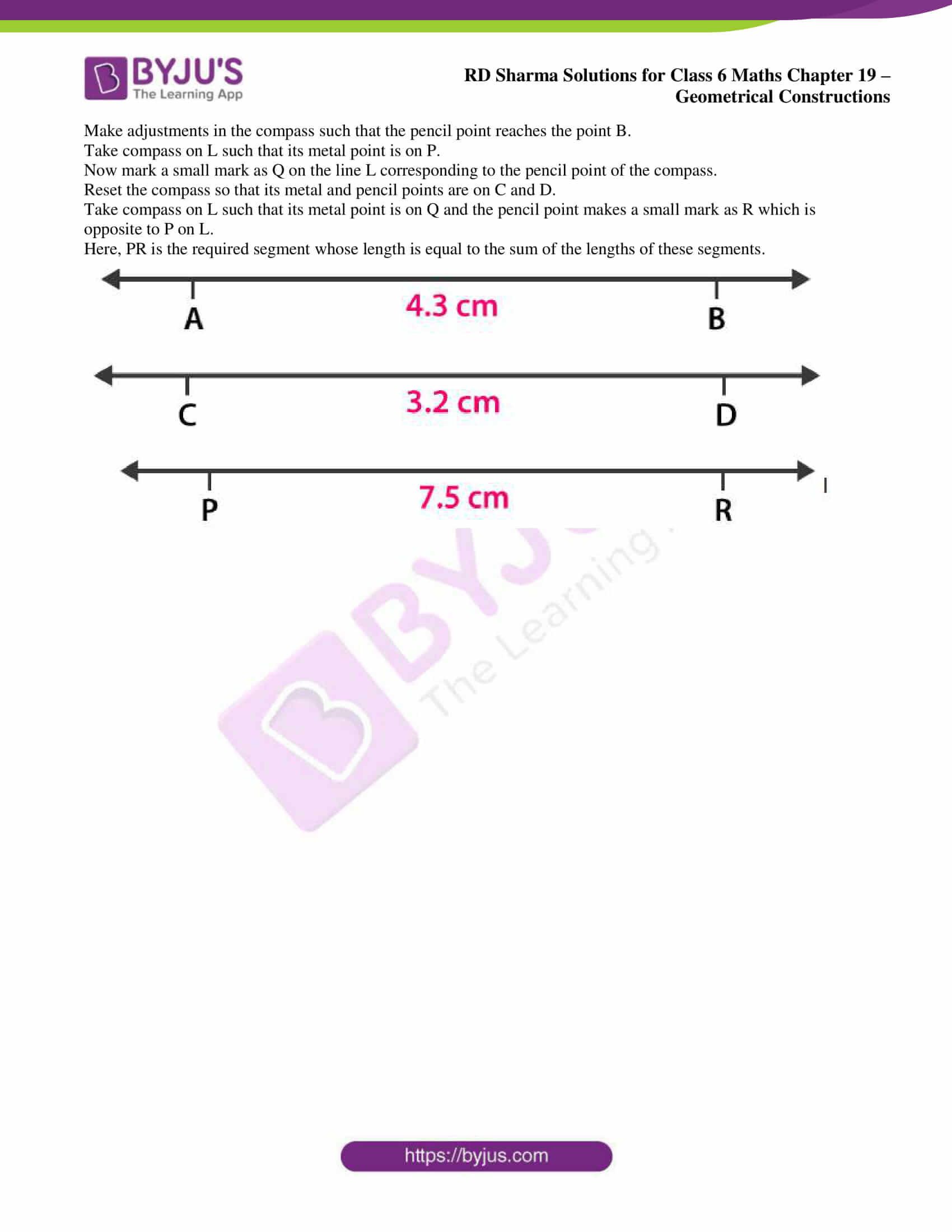### Exercise 19.1 page: 19.2

1. Construct line segments whose lengths are:

(i) 4.8 cm

(ii) 12 cm 5 mm

(iii) 7.6 cm

Solution:

(i) 4.8 cm

Construct a line L on a paper and mark A on it.

Now place the metal point of the compass at zero mark of the ruler.

Make adjustments in the compass such that the pencil point is at 4.8 cm mark on the ruler.

Take compass on L such that its metal point is on A.

Now mark a small mark as B on L which is corresponding to the pencil point of the compass.

Here, AB is the required line segment of length 4.8 cm.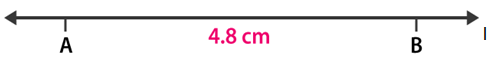(ii) 12 cm 5 mm

Construct a line L on a paper and mark A on it.

Now place the metal point of the compass at zero mark of the ruler.

Make adjustments in the compass such that the pencil point is at 5 small points from the mark of 12 cm to 13 cm on the ruler.

Take compass on L such that its metal point is on A.

Now mark a small mark as B on L which is corresponding to the pencil point of the compass.

Here, AB is the required line segment of length 12 cm 5 mm.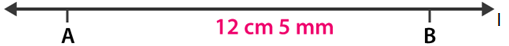(iii) 7.6 cm

Construct a line L on a paper and mark A on it.

Now place the metal point of the compass at zero mark of the ruler.

Make adjustments in the compass such that the pencil point is at 6 small points from the mark of 7 cm to 8 cm of the ruler.

Take compass on L such that its metal point is on A.

Now mark a small mark as B on L which is corresponding to the pencil point of the compass.

Here, AB is the required line segment of length 7.6 cm.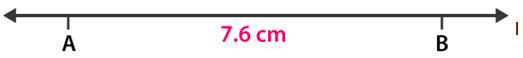2. Construct two segments of length 4.3 cm and 3.2 cm. Construct a segment whose length is equal to the sum of the lengths of these segments.

Solution:

With the help of compass and ruler, construct AB and CD of lengths 4.3 cm and 3.2 cm.

Construct a line L and mark P on it.

Now place the metal point of the compass at zero mark of the ruler.

Make adjustments in the compass such that the pencil point reaches the point B.

Take compass on L such that its metal point is on P.

Now mark a small mark as Q on the line L corresponding to the pencil point of the compass.

Reset the compass so that its metal and pencil points are on C and D.

Take compass on L such that its metal point is on Q and the pencil point makes a small mark as R which is opposite to P on L.

Here, PR is the required segment whose length is equal to the sum of the lengths of these segments.